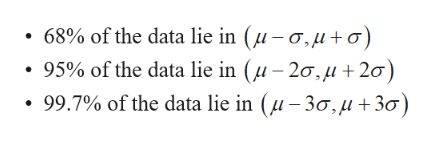# SAT Math scores have a bell-shaped distribution with a mean of 515 and a standard deviation of 114. Use the empirical rule a) What percentage of SAT scores is between 401 and 629b) What percentage of SAT score is less than 401 or greater than 629?

Question
33 views

SAT Math scores have a bell-shaped distribution with a mean of 515 and a standard deviation of 114. Use the empirical rule

a) What percentage of SAT scores is between 401 and 629

b) What percentage of SAT score is less than 401 or greater than 629?

check_circle

Empirical rule:

If the distribution is bell shaped, the Empirical Rule can be used. The Empirical ru...help_outlineImage Transcriptionclose68% of the data lie in (μ- σ,μ + σ) . 95% of the data lie in (μ-2σ, μ + 2σ) . 99.7% of the data lie in (μ- 3σ,μ + 3σ ) fullscreen

### Want to see the full answer?

See Solution

#### Want to see this answer and more?

Solutions are written by subject experts who are available 24/7. Questions are typically answered within 1 hour.*

See Solution
*Response times may vary by subject and question.
Tagged in

### Statistics